# MCAT Physical : Reduction Potentials

## Example Questions

### Example Question #1 : Reduction Potentials

Consider the half reaction below.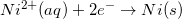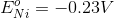Which of the following statements is true about the oxidation of nickel?

The oxidation of nickel has a potential of 0.23V

It is not possible to oxidize nickel

None of these are true

The oxidation of nickel has a potential of 0V

The nickel will gain electrons when oxidized

The oxidation of nickel has a potential of 0.23V

Explanation:

Reduction potentials are typically provided in table form. Since the oxidation potential of an element is the negative of the reduction potential, it can be determined by viewing a reduction table.

Since the reduction potential for nickel is given as –0.23V, we can conclude that the oxidation potential for nickel is 0.23V.

### Example Question #2 : Reduction Potentials

The standard reduction potentials for iron and nickel are as follows: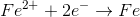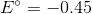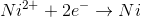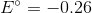If a galvanic cell is made using these two metals, what is the standard voltage for the cell?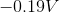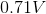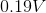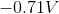Explanation:

A galvanic cell is spontaneous, meaning that there will be a positive cell voltage. In order for this to take place, the more negative reduction potential must be flipped so that the metal is oxidized.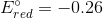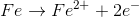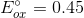Adding these values together gives a standard cell voltage of.